RS Aggarwal Class 8 Solutions Chapter 8 - Linear Equations

RS Aggarwal Class 8 Chapter 8 - Linear Equations Solutions Free PDF

Linear equations is one of the most important topics of CBSE class 8. Learn the concepts of linear equations by solving questions from RS Aggarwal for class 8. Learning the concepts linear equations will help the student to solve the questions based on linear equations in one variable and linear equations in two variables. Students also refer to RS Aggarwal class 8 solutions chapter 8 linear equations for the detailed answer to RS Aggarwal questions.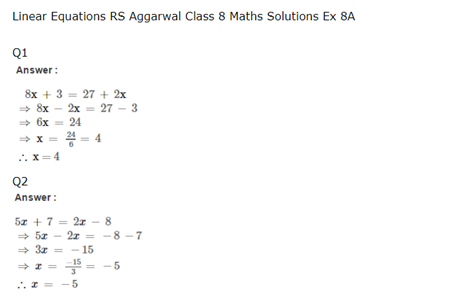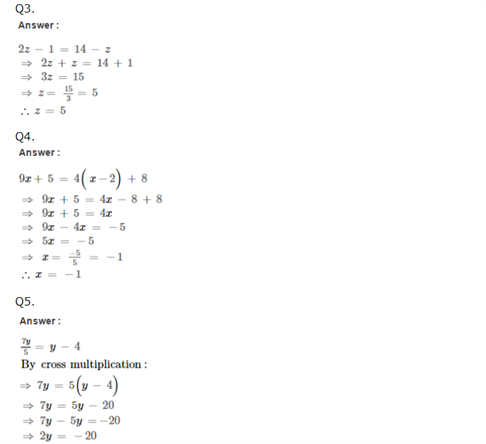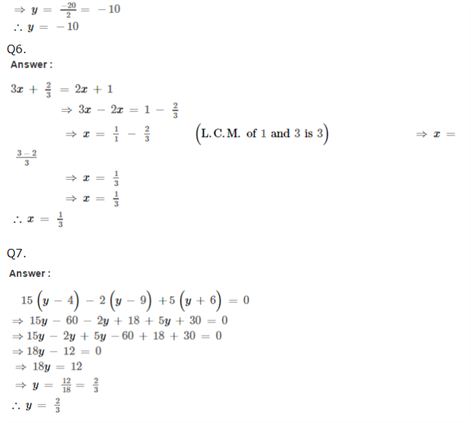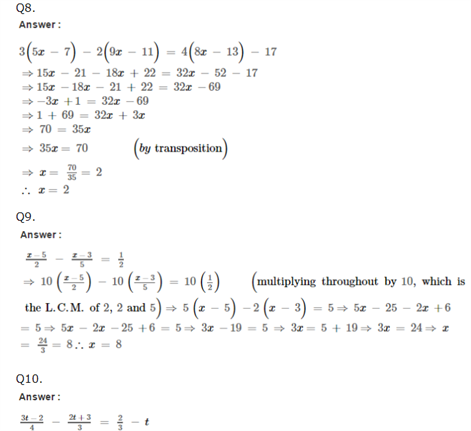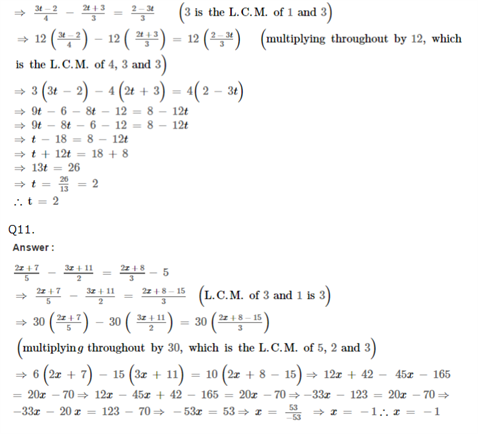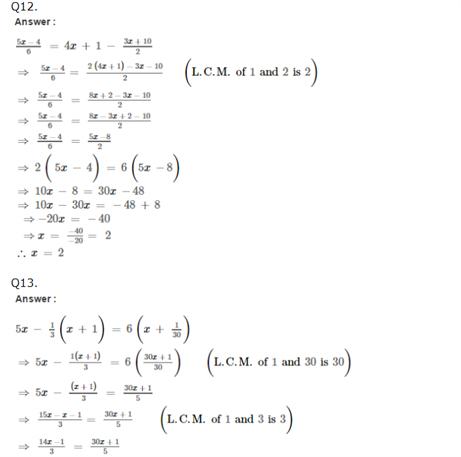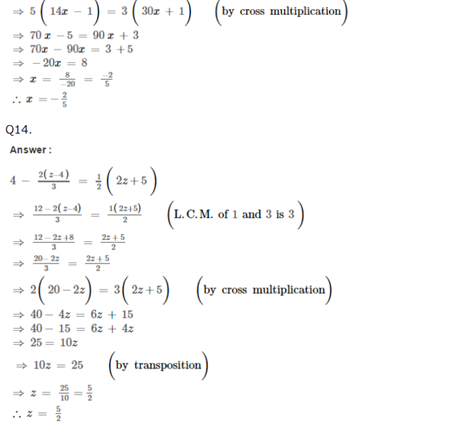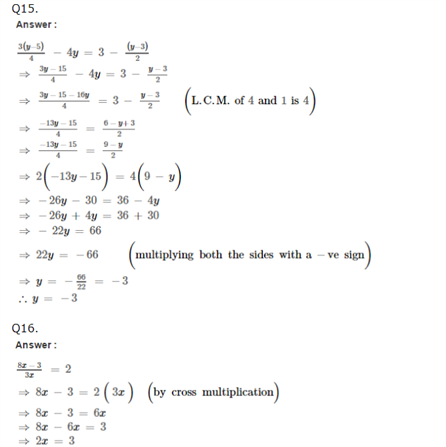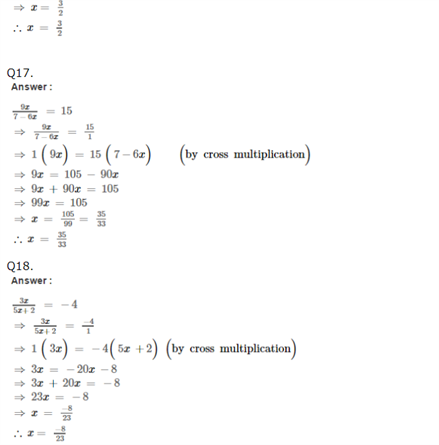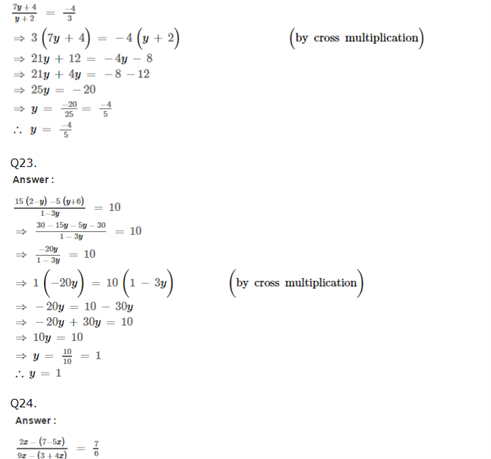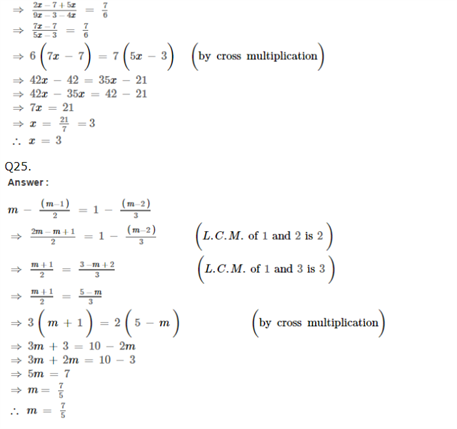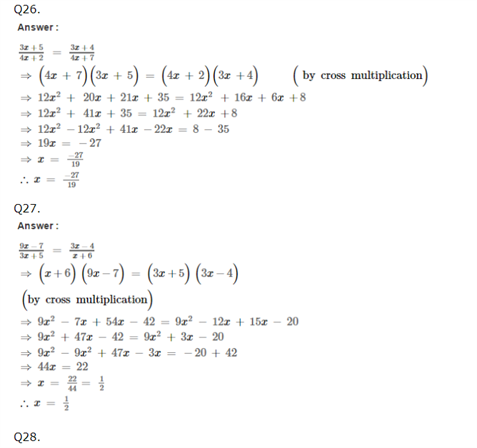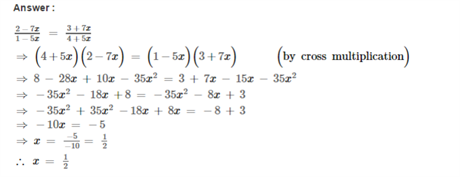Practise This Question

In a triangle, the sum of the lengths of any two sides must be less than the third side.